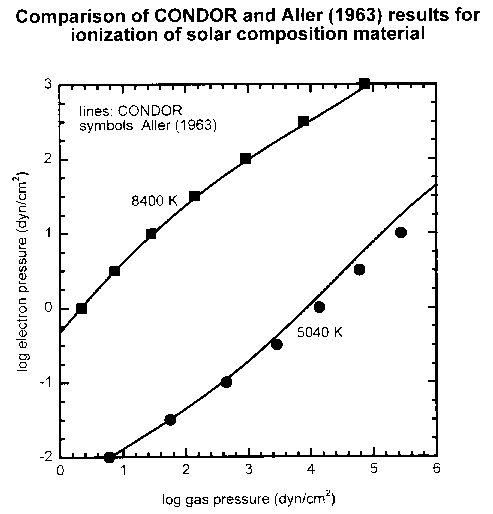# Stellar Atmospheres

CONDOR – Applications

Astronomers are interested in the extent of ionization of the elements in stellar atmospheres as a function of bulk composition (e.g., deviation from solar composition), temperature, and pressure.  Aller (1963) gives a discussion of computations of ionization using Boltzmann statistics and the Saha equation and also presents tabular data and a graph based on computations using the Goldberg et al. (1960) solar elemental abundances.  Below we give a comparison of CONDOR results with results from Aller (1963). This is presented graphically using the format in his book where the electron pressure is plotted as a function of the gas pressure on a logarithmic scale.  The pressures are given in dyn/cm2 and the two temperatures shown (5040 K and 8400 K) correspond to theta = 1 and theta = 0.60 respectively.  Note that 106 dyn/cm2 = 1 bar.References

1. Aller, L. A. 1963.  The Atmospheres of the Sun and Stars, Ronald Press, New York.